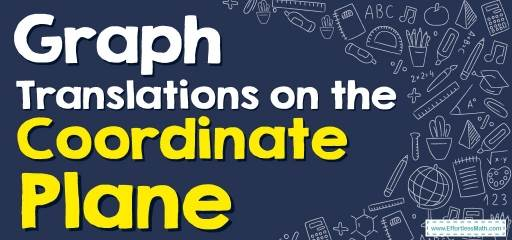# How to Graph Translations on the Coordinate Plane?

This article teaches you how to Graph Translations on the Coordinate Plane in a few simple steps.## Step by step guide to Graph Translations on the Coordinate Plane

Translation on the coordinate plane is sliding a point or figure in any direction without any changes in size or shape. In fact, during translation, the coordinates of the vertices of a figure or point change, and they slide left or right, up, or down without changing size or shape.

Translations in a coordinate plane can be described by this coordinate notation: $$(x, y)→(x+a, y+b)$$, where $$a$$ and $$b$$ are constants. Each point shifts $$a$$ units horizontally and $$b$$ units vertically. Note that to graph a translation, you should perform the same change for each point.

### Translations on the Coordinate Plane – Example 1:

Translate triangle IGH $$-2$$ units in the $$x-$$direction and $$-3$$ units in the $$y-$$direction.

Solution:

First, write the original coordinates of the points:

$$I=(-2, 4)$$ $$G=(1, 2)$$ $$H=(4, 3)$$

Use this coordinate notation for translating each point: $$(x, y)→(x+a, y+b)$$

$$a=-2$$, $$b=-3$$, then: $$(x, y)→(x-2, y-3)$$

Then: $$I^\prime=(-4, 1)$$ $$G^\prime=(-1, -1)$$ $$H^\prime=(2, 0)$$

Now, find new points on the coordinate plane and graph the new triangle by $$I^\prime, G^\prime, H^\prime$$ coordinates.

### Translations on the Coordinate Plane – Example 2:

Graph the image of the figure using the transformation given.

Translation:  $$4$$ units left and $$3$$ units up.

Solution:

First, write the original coordinates of the points:

$$I=(3, 0)$$ $$G=(2, -4)$$ $$H=(5, -2)$$

Use this coordinate notation for translating each point: $$(x, y)→(x+a, y+b)$$

$$4$$ units left →$$a=-4$$

$$3$$ units up →$$b=3$$

Then: $$I^\prime=(-1, 3)$$ $$G^\prime=(-2, -1)$$ $$H^\prime=(1, 1)$$

Now, find new points on the coordinate plane and graph the new triangle by $$I^\prime, G^\prime, H^\prime$$ coordinates.

## Exercises for Translations on the Coordinate Plane

### Graph the image of the figure using the transformation given.

1.Translation: $$5$$ units right and $$2$$ unit up.

2.Translation: $$3$$ units left and $$5$$ unit up.

### What people say about "How to Graph Translations on the Coordinate Plane? - Effortless Math: We Help Students Learn to LOVE Mathematics"?

No one replied yet.

X
30% OFF

Limited time only!

Save Over 30%

SAVE $5 It was$16.99 now it is \$11.99